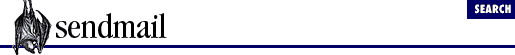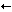#Chapter 38Rule-Set Testing with -bt## 38.4 Show an Item

Beginning with V8.7 sendmail, two rule-testing commands are available: the `=S` command displays all the rules in a given rule set, and the `=M` command displays all the delivery agents. Both display their items after the configuration has been read. Thus in the case of rules, all the macros will have already been expanded.

Both are triggered by the leading `=` character. If nothing follows the `=`, this usage message is printed:

`Usage: =Sruleset or =M`

If any character other than `S` or `M` follows the `=` character, the following error is printed:

`Unknown "=" command =bad character here`

### 38.4.1 Show Rules in a Rule Set with =S

The `=S` rule-testing command causes sendmail to show all the rules of a rule set. The form of this command looks like this:

`=S`ruleset``

Optional whitespace may separate the `ruleset` from the `S`. The `ruleset` may be a number or a symbolic name (see Section 29.1.2, "Rule-Set Names"):

```=S0a number
=SMyrulea name```

Note that although macros may be used in defining rule sets (see Section 29.1.4, "Macros in Rule-Set Names"), they may not be used with the `=S` command:

```> =S\$X
invalid ruleset name: "\$X": No such file or directory
Undefined ruleset \$X
>```

One use for the `=S` command is to determine why a rule set is not behaving as expected. Consider a rule set 1 that is intended to rewrite all sending addresses so that the local hostname makes the message appear as though it came from the mail hub machine. Suppose that, when testing, you send an address through that rule but it comes out unchanged:

```> `1 bob@localhost`
rewrite: ruleset   1   input: bob @ localhost
rewrite: ruleset   1 returns: bob @ localhost
>```

Puzzled, you look at the actual rule with the `=S` rule-testing command:

```> `=S1`
R\$* < @ \$=w > \$*                \$@ \$1 < @ mailhub . our . domain > \$3
>```

Aha! Rule set 1  expects the host part of the address to be surrounded by angle brackets! Knowing this, you run the address through the rule again, this time using angle brackets, and it succeeds:

 For the sake of the example we limited this rule set to a single rule. Most rule sets will have many rules.

```>  `1 bob<@localhost>`
rewrite: ruleset   1   input: bob < @ localhost >
rewrite: ruleset   1 returns: bob < @ mailhub . our . domain >
>```

### 38.4.2 Show Delivery Agents with =M

The `=M` rule testing command causes sendmail to print its list of delivery agents. This command takes no argument. Note that in the following example the lines are wrapped to fit on the page:

```> `=M`
mailer 0 (prog): P=/bin/sh S=10/30 R=20/40 M=0 U=0:0 F=DFMelosu L=0 E=\n T=X-Unix
/rfc822/smtp A=sh -c \$u
mailer 1 (*file*): P=[FILE] S=0/0 R=0/0 M=0 U=0:0 F=DEFMPlosu L=0 E=\n T=DNS/RFC8
22/X-Unix A=FILE
mailer 2 (*include*): P=/dev/null S=0/0 R=0/0 M=0 U=0:0 F=su L=0 E=\n T=<undefine
d>/<undefined>/<undefined> A=INCLUDE
mailer 3 (local): P=/bin/mail S=10/30 R=20/40 M=0 U=0:0 F=/5:@ADFMlmnrsw| L=0 E=\
n T=DNS/RFC822/X-Unix A=mail -d \$u
mailer 4 (smtp): P=[IPC] S=11/31 R=21/21 M=0 U=0:0 F=DFMXmu L=990 E=\r\n T=DNS/RF
C822/SMTP A=IPC \$h
mailer 5 (esmtp): P=[IPC] S=11/31 R=21/21 M=0 U=0:0 F=DFMXamu L=990 E=\r\n T=DNS/
RFC822/SMTP A=IPC \$h
mailer 6 (smtp8): P=[IPC] S=11/31 R=21/21 M=0 U=0:0 F=8DFMXmu L=990 E=\r\n T=DNS/
RFC822/SMTP A=IPC \$h
mailer 7 (relay): P=[IPC] S=11/31 R=61/61 M=0 U=0:0 F=8DFMXamu L=2040 E=\r\n T=DN
S/RFC822/SMTP A=IPC \$h```

This output is the same as that produced with the `-d0.15` debugging switch (see Section 37.5.4, -d0.15). The individual items in each line are explained in Chapter 30, Delivery Agents.

Internally, the `=M` rule testing command calls printmailer() to print the contents of each delivery agent.